# The Octagonal Antiprism

The octagonal antiprism is a 3D uniform polyhedron bounded by 18 polygons (2 octagons and 16 triangles), 32 edges, and 16 vertices.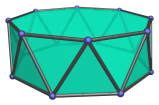The height of an octagonal antiprism with an edge length of 2 is:

2√((1 + √2)(√(1 + 1/√2) - 1))

which is approximately 1.720591.

The square cupola (J4) can be pasted with an octagonal antiprism to produce a gyroelongated square cupola (J23). Adding a second cupola produces the gyroelongated square bicupola (J45).

## Projections

Here are some views of the octagonal antiprism from various angles:

Projection Envelope Description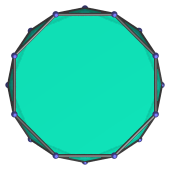Regular hexadecagon

Parallel projection centered on octagonal face.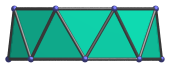Trapezium

Parallel projection parallel to square faces and a pair of triangles.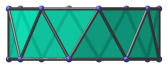Rectangle

11.25° side view.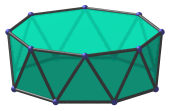Nonagon

Parallel projection centered on vertex.

## Coordinates

The Cartesian coordinates of the octagonal antiprism, centered on the origin and having edge length 2, are:

• (±1, ±(1+√2), H)
• (±(1+√2), ±1, H)
• (0, ±√(4+2√2), -H)
• (±√(4+2√2), 0, -H)
• (±√(2+√2), ±√(2+√2), -H)

where H = √((1 + √2)(√(1 + 1/√2) - 1)), or approximately 0.860296, is half the height of the antiprism.

Last updated 17 Jun 2019.